Next: 3.2 Interaction of Dopants Up: 3. Diffusion Phenomena in Previous: 3. Diffusion Phenomena in

# 3.1 The Physics of Diffusion

Diffusion is the kinetic process that leads to the homogenization, or uniform mixing, of the chemical components in a phase. Although mixing in a fluid (liquid or gas) may occur on many length scales, as induced by macroscopic flow, diffusive mixing in solids, by contrast, occurs only on the atomic or molecular level. As time increases, the extent of homogenization by diffusion also increases, and the length scale over which chemical homogeneity persists within a phase gradually extends to macroscopic distances.

In the silicon process technology, depending on the complexity of the model, dopant redistribution does not only include dopant atoms itself, but also point defects. The point defects can be divided into simple and extended point defects.

Additionally, the presence of the large number of the charged dopant atoms causes an electrical field in the inside of the wafer, which reversely influences the diffusion of dopants.

The continuum-based diffusion models are successfully applied in many process simulation programs . These models use the so-called methodology of diffusion-reaction equations, which was shown to be very efficient for the numerical simulation of the diffusion phenomena. The method itself can be generalized to design a set of coupled partial differential equations from some assumption about possible reactions between intrinsic point defects and dopants.

Principal ingredients of the diffusion-reaction models are: Fick's diffusion laws, electrical field enhanced diffusion, and species generation and recombination theory. In the following we will briefly discuss and illustrate each of these basic concepts.

Fick's diffusion laws
The laws of diffusion are mathematical relationships which relate the rate of diffusion to the concentration gradient for net mass transfer. Such laws are considered to be phenomenological. We define vector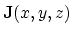to be a mass flux of the diffusing component. In Cartesiancoordinates, with the unit coordinates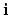,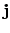and, the mass flux may be written as a vectorial sum: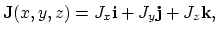(3.1)

The individual flux magnitudes appearing in (3.1) can be expressed by component equations through Ficks's first law:(3.2)

where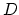denotes the diffusion coefficient and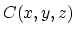is the concentration of the diffusing component.
By imposing a mass conservation on the diffusing system we obtain Ficks's second law in the form: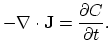(3.3)

The interpretation of (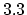) is clear: If there is a converging flow of species at a point, so that, the concentration rises with time; if there is a divergent flow, so that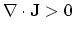, the concentration falls.

Influence of the Electrical field
We consider a single charged particle moving in the electrical field with potential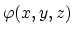. The gradient of this potential describes the force on the particle ,(3.4)

where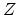is the valence of the charged species. A potential gradient tends to produce a flux of the species, and this flux must be added to that produced by the concentration gradient to arrive at the equation for the total flux. It is found empirically that a potential gradient gives rise to a mean diffusion velocity for the affected atoms. This fact is mathematically expressed by the equation,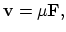(3.5)

in which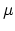is called mobility. The force gives rise to a steady-state velocity instead of continuing acceleration.

In applying a potential gradient instead of concentration gradient we are simply replacing one small force with another. Thus is plausible that the mobility is proportional to the diffusion coefficient. This fact is expressed by Einstein's relationship,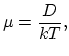(3.6)

where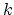is the Boltzmann constant. The flux that results in a homogenous system is thus,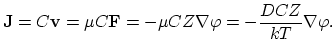(3.7)

Combining the last equation with Fick's diffusion law we obtain a partial differential equation describing the behavior of the charged particle in the concentration and electrical gradient field,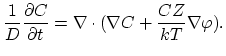(3.8)

Electric fields arise from the large differences in diffusion constants of the ionized dopants and the charge carriers associated with them . The charge carriers would ordinary tend to diffuse away faster then the ions, thus creating a space charge. The electric field originating from this space charge acts on the ions and charge carriers to counteract the separation.

Generally, in the case of non-uniform concentrations of dopants, the electric field calculation is based on third Maxwell's equation,

 div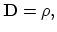(3.9)

where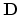is the electric displacement vector and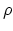the space charge concentration. The electric displacement vector is related to the electric field vectorand thus to the electrostatic potential,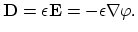(3.10)

Finally, under the assumption of complete ionization, (3.9) takes the form ,

 div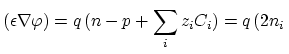sinh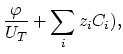(3.11)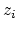denotes the electron charge associated with the defects and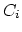is their concentration.

Assuming local charge neutrality,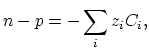(3.12)

it can be shown that electron and hole concentration is expressed by ,(3.13)

where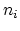is intrinsic carrier concentration (see Section 3.3.1).

Species generation and recombination theory
During the diffusion process species may arrange in pairs with species of another type and thereby the new species are created. Let we take three species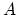,and, whereas the third one is constructed by pairing of first and second. This chemical reaction can be symbolically displayed as ,(3.14)

The intensity of these reaction is expressed by a rate coefficientAlso the reverse process is thinkable, dissolution of the compound speciesinto the original speciesand,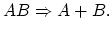(3.15)

The rate coefficient of the reverse reaction is. For these two reactions we can also define the rate of reaction by,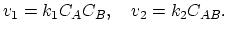(3.16)

We say that the reactions are in global thermal equilibrium if everywhere is fullfiled,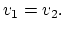(3.17)

A thermal dependence of the reaction rates is always assumed, and with taking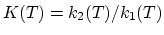, (3.17) can be written in the form of the law of mass action,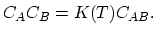(3.18)

However, if the reactions are not in global thermal equilibrium, there is obviously a change in concentration of each species and the following equations hold,(3.19)Relations (3.19) are important for advanced diffusion models.Next: 3.2 Interaction of Dopants Up: 3. Diffusion Phenomena in Previous: 3. Diffusion Phenomena in

H. Ceric: Numerical Techniques in Modern TCAD